Nonextensive Electrical and Thermostatistical Parametric Dependency on Temperature and Tsallis Factor by the Formulation of Q-Exponential Integrals | OMICS International
Journal of Physical Mathematics

# Nonextensive Electrical and Thermostatistical Parametric Dependency on Temperature and Tsallis Factor by the Formulation of Q-Exponential Integrals

Banerjee Avinab*

Department of Physics, Birla Institute of Technology, Mesra, India

*Corresponding Author:
Banerjee Avinab
Researcher, Department of Physics
Birla Institute of Technology
Mesra, India
Tel: 919855678465
E-mail: [email protected]

Received Date: July 03, 2017; Accepted Date: August 30, 2017; Published Date: September 04, 2017

Citation: Banerjee Avinab (2017) Nonextensive Electrical and Thermostatistical Parametric Dependency on Temperature and Tsallis Factor by the Formulation of Q-Exponential Integrals. J Phys Math 8: 244. doi: 10.4172/2090-0902.1000244

Copyright: © 2017 Banerjee Avinab. This is an open-access article distributed under the terms of the Creative Commons Attribution License, which permits unrestricted use, distribution, and reproduction in any medium, provided the original author and source are credited.

Visit for more related articles at Journal of Physical Mathematics

#### Abstract

The paper emphasizes an approach to solve complex q-exponential integrals by an implication of Binomial expansion along with some specific conditions on the integral limits and sets a relation between the limits which were used such as the maximum energy of the electrons and the chemical potential. The integral results which yielded the average energy and the effective electron concentration which proved out to be essential in order to calculate the average velocity of the electrons and the electrical conductivity of a metallic wire while taking consideration of the Drude’s model. The dependency on temperature of these parameters with the variation of Tsallis factor renders anomalous results depicting the Nonextensive Critical Temperature value to be 20 K and its importance has been mentioned in this paper along with the dependency of these parameters on certain regions consisting of Tsallis factor which follows subextensivity and superextensivity and infers a point known to be as Maximum Entropy Limiting Factor.

#### Keywords

q-Exponential integrals; Nonextensive electrical conductivity; Nonextensive root mean square velocity; Nonextensive average energy; Nonextensive critical temperature value; Maximum entropy limiting factor

#### Introduction

Nonextensive statistical mechanics is one of the contemporary pillars of physics which was formulated in the year 1988 in the famous Journal of Statistical Physics known to be as “Possible generalization of the Boltzmann-Gibbs statistics” . This new era of statistical mechanics is being used to classify several kinds of entropy which exists in the system, to calculate it and to find the possibility of its reduction inside the system to some extent. This branch extends the empire of Statistical Mechanics while validating its applicability and its interconnectivity between metaphysics, mesoscopic physics and macroscopic physics. This branch is based upon the theory of probabilities i.e., CLT (Central Limit Theorem) . Based upon the CLT the Fermi-Dirac distribution function with an introduction of the Tsallis factor q [1-3] is given by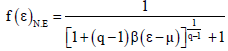The effective electron concentration has been calculated by the integral of the product of the distribution function along with the density of states of the electron gas forming a q-exponential integral. The density of states has been formulated by considering a sphere of volume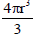which is known to be as the momentum sphere. Subsequently, the average energy of the electron gas also yields in the form of a q-exponential integral which was analyzed by Tsallis and Brigatti . Solving the two simultaneous q-exponential integrals conditionally by using Binomial expansion, integration by substitution and assuming conditions within the limits of the integral being formulated with respect to the cold temperature values and the hot temperature values accordingly yields us the value of the average energy of the electron gas inside the conductor which should be 60% to that of the Fermi energy [4,5] value at q=1 (standard behavior). The average velocity of the electron gas inside the conductor exceeding the relativistic speed limit for lower temperature values and for the values of q residing at the regions of subextensivity  and superextensivity  has been shown in the curve plotted between the root mean square velocity of the electron gas and the Tsallis factor.

The electrical conductivity of the metallic wire was calculated by the Drude’s equation of electrical conductivity given by the equation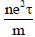[6,7] which can be further simplified in the terms of drift velocity and mean-free path length to be as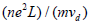[6,7] where the drift velocity is the average velocity acquired by the electrons with a subjection to an external excitation field (electric field). The variation of the electrical conductivity with respect to the Tsallis factor pertain the inverse relation existing between the electrical conductivity and the average velocity of the electron gas inside the conductor. Diminishment of the non-linearity errors have been found in the curve plotted between the electrical conductivity and temperature. The paper also recites the significance of this theory while building a new scope in the area of superconductivity. This has been explained by the persistence of a signal (information) inside a conductor at lower values of temperature. The paper also accounts with an increment or decrement of entropy within the system according to the regions of subextensivity and superextensivity. As, we know that the Lyapunov exponent diminishes in a non-extensive domain as the thermodynamic limit approaches to infinity [1-3] in an ergodic system [1-3]. The molecular chaos theory [1-4] is applicable to this case because a meniscus amount of scattering occurs due to the defects present in the lattice at the lower temperature values. Weak chaos of the molecules does occur along with the long range interactions of the colder ions which are generated due to a temperature residing across to the absolute zero.

#### Calculation of Density of States

The density of states is said to be as the number of possible states which can occupied by the electron per unit volume of the space which is available in the system [6,8]. This can be calculated by the consideration of a momentum sphere which is three dimensional and contains a volumein the three dimensional space [6,8]. The electron cloud can fit inside the sphere in any manner and even occupying the same volume as that of the momentum sphere of radius ‘k’ as shown in Figure 1.

The above concentric circles depict the three dimensional momentum sphere by which we are ought to calculate the density of states or the number of available states which can be occupied by the carriers of the conductor [6,8].

In this context it is the volume of the sphere inside which the total number of the carriers of the conductor can be fitted [6,8]

As, we know that the volume of the sphere is given by. Therefore, we can proclaim the total number of available states to be as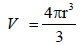(1)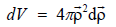(2)

(Rate of change of volume yields surface area which is the density of states of the depicted sphere).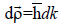(3)

(Rate of change of momentum in the equation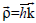Using equations (1), (2) and (3) we get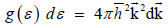(4)

We know that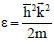. Therefore,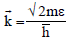and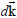is calculated accordingly in equation (5)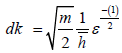(5)

putting equation (5) in (4) substituting the values of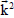andwe get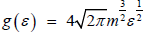(6)

The above expression of the density of states [5,6,8] is going to be used in calculating the parameters which are being mentioned above.

#### Effective Carrier Concentration

The effective carrier concentration is the total number of carriers (electrons in a metal) residing inside the conductor. As, we know that it can be calculated by the integration of the product of the density of states along with the distribution function of the carriers [6,8] as follows: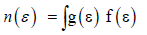(7)

The distribution function determines the probability of occupancy and the vacancy of the carriers within that total number of available states [6,8]

The Fermi-Dirac distribution function has been taken into consideration since we are dealing with a group of fermions inside the vicinity of the conductor known to be as the electron cloud or electron gas. With an introduction of the Tsallis Factor ‘q’ the distribution function becomes to be as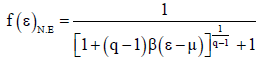(8)

Equation (8) can be expressed in the terms of probability as being mentioned previously as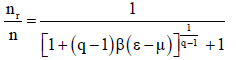(9)

Where nr is the number of carriers acquiring the chemical potential and intending to reach the maximum energy level, n is the total number of carriers available in the non extensive domain which is said to be fixed [2,3] ε is the energy associated with the particles which they are going to acquire in order to surpass the, chemical potential μ and ‘q’ is the Tsallis entropy factor.

Therefore,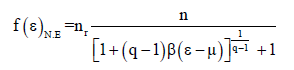(10)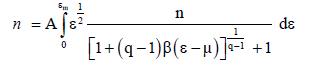(11)

by using equation (6) and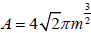obtained in equation (6).

Subsequent deduction of equation (9) becomes to be as –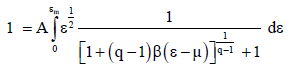(12)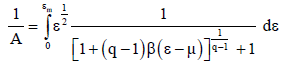(13)

Solving integrals like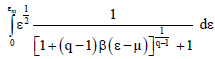are quite difficult. Henceforth, we need to perform certain substitutions, assumptions and need to perform Binomial Expansion.

Substituting for unity we get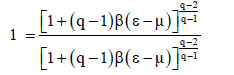(14)

(In order to make the numerator and the denominator do consist of equal terms for the binomial expansion).

Let ε-μ=x (15)

Calculating the rate of change of the variables on both the respective sides we get

dε=dx (16)

The equation becomes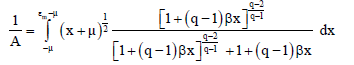(17)

Expanding the two expressions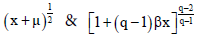by Binomial Expansion by assuming two conditions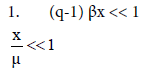By the Binomial expansion of the terms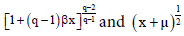we obtain the expression to be as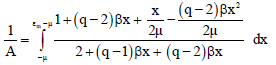(18)

Successive integration by substitution we get an expression to be as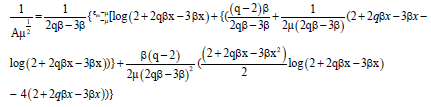(19)

Applying the condition numbered to be as 2 on the equation (19) we get εm=2μ and as a result of this we get new limits to our solution of the integral which is highly sophisticated to be (-μ) to μ. The final expression we get to be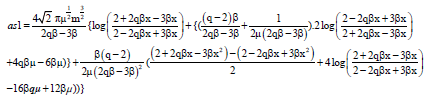(20)

#### Average Energy of the Electrons

The average energy is the integration of the product of the total energy along with the effective carrier concentration . A similar type of solution can be obtained for the average energy which can be calculated by the equation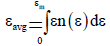(21)

Using the Nonextensive Fermi-Dirac distribution function which is being mentioned in the section above

Therefore, similar to that of the previous section the distribution function can be expressed in terms of probability as follows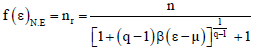(22)

Incorporating the logic of equation (1) along with equation (22) in equation (21) we get an expression to be as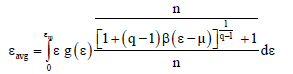(23)

Substituting the value of nr in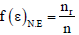we get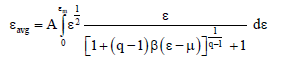(24)

By using the analogy of equation (6) in order to put the value of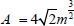Subsequent deduction of equation (24) becomes to be as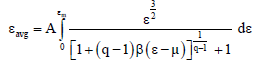(25)

Similar to that section of the calculation of the effective carrier concentration which yielded in such complex integrals like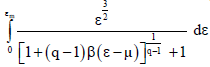are very difficult to solve and require certain substitutions, assumptions and binomially expanding the power tailed expression of the denominator part.

Subsequent substitution for unity in order to make the numerator and the denominator do consist of equal terms for the binomial expansion.

Let ε-μ=x (26)

Calculating the rate of change of the variables on both the respective sides we get

dε=dx (27)

The equation becomes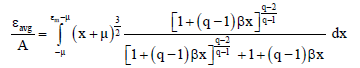(28)

Similar expansion of the two expressions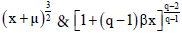by Binomial Expansion with the assumption of two conditions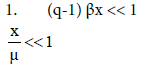By the Binomial expansion of the terms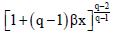and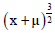we obtain the expression to be as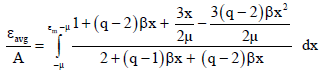(29)

Successive integration by substitution we get an expression to be as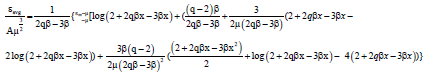(30)

Applying the condition numbered to be 2 on the equation (30) we get εm = 2μ and as a result of this we get new limits to our solution of the integral which is highly sophisticated to be (-μ) to μ. The final expression we get to be as

Now dividing equation (31) by equation (20) we get an expression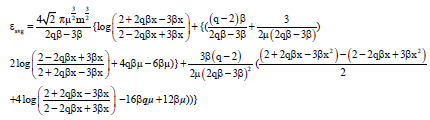(31)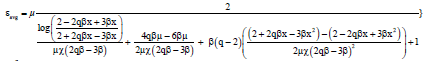(32)

where,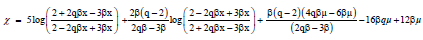(33)

#### Average Velocity

The average velocity of the electrons is being calculated in order to find the electrical conductivity of the metallic wire.

We know that [7,8]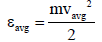(34)

Further deduction in order to get an expression for vavg we get an expression to be as [7,8]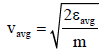(35)

Incorporating equation (32) in the equation (35) we yield the value of the average velocity to be as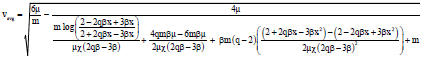(36)

Where χ is same as that of the equation (33).

#### Electrical Conductivity

The electrical conductivity of the metallic wire can be defined as the ratio of the current density to that of the applied external electric field [6,7]. The versatility of the Drude’s model in order to unravel the occult nature of the electron cloud (electron gas) has been taken into consideration and by using the Drude’s equation [6,7] to be as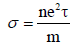(37)

The equation above can be deduced further by substituting the value of the relaxation time [6,7] which is given to be as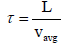(38)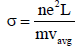(39)

Incorporating equation (36) in the equation (39) we get the yield which we were intended to find that is the electrical conductivity to be as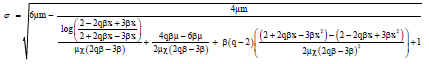(40)

Where χ is same as that of the equation (33).

#### Results and Discussions

Figure 2 infers that when ‘q’=0 there is an increment in the average energy of the electrons starting from (-5.54)× 10-6J at 20 K which means that when there is no entropy factor which is required to regulate the entropy of a system, the entropy of a system tends to increase by energizing the electrons which possessed a minimum amount of energy at lower temperature values. It also renders us the physical significance of the non-existence of any regulation in the entropy of the system. But, actually there exists a regulation in the entropy which is present in a considerable amount because the region ‘q’<1 is known to be as the region of superextensivity . When ‘q’=1 the system follows extensivity and is subjected to tremendous amount of disturbances and as a result it yields an average value 60% to that of the Fermi energy (Chemical Potential) . The temperature at which the average energy tends to increase is quite low and it is non-feasible in nature but since, we are using a regulatory parameter to decrease the entropy of the system several kinds of such anomalous characteristic can be observed. This low anomalous temperature value is known to be as ‘Non–extensive Transition Temperature’. We are able to observe that for the other values of q i.e., when ‘q’=0.25, ‘q’=0.6 and even when ‘q’=1 (follows extensive characteristics) [2,3] the electrons do posses a value of the average energy i.e., which is greater than that when ‘q’ =0. This is because we are traversing towards the direction of the region of ‘subextensivity’ i.e., when ‘q’>1 . The only plots which are visible to us are that of ‘q’=0 (superextensivity), ‘q’=2 and ‘q’ =1.15(subextensivity). The entropy of a system is decreased at a larger extent in the region of subextensivity as compared to the region of superextensivity that is why we observe an increasing value of the average energy. That is why the diamond structure appears earlier as compared to the blue line at the non-extensive transition temperature.

According to the Figure 3 depicted above the average velocity (drift velocity) [10,11] of the electrons is higher for ‘q’=0.25 and ‘q’=2 that completely specifies superextensivity  and subextensivity . The speed of light or the relativistic limit is surpassed at these values of ‘q’ at lower value of the temperatures by the regulation of the entropy diminishes at a higher extent at a higher value of ‘q’ i.e ‘q’=2 which happens due the entropy factor ‘q’ regulating upon the electrons of the system making them move freely with very lesser amount of scattering phenomenon which is due to the defects present in the lattice structure of the metal to occur and as a result increases the phase velocity of the electrons. The increment of the phase velocity of the carriers is due to the lesser interaction of it with its nearby electrons or ions which can generate a certain amount of potential. If any such chaos occurs it would be weaker (weak chaos)  and the Lyapunov exponent vanishes relaxation time τ decreases as a result [1,3,9]. This is due to the regulatory action of the Tsallis entropy factor ‘q’ acting upon the distribution function of the class of electrons present in the domain and each and every one of them gets affected. As, a result the electron does posses an average velocity nearer to the relativistic limit. The greater the value of ‘q’ more the inhibition of an electron takes place making them to scatter and collide weakly. The meniscus amount of the scattered electrons do posses tremendous amount of acquired energy from ‘q’ which has altered its phase velocity significantly interacts with the group of non scattered electrons which already does possess a phase velocity approximate to the relativistic speed limit. This weak interaction inhibits some feeble but sumptuous amount of energy to the non scattered group of electrons. When there is the existence of cold temperature values then there would not be that amount of external disturbances which would resist the path of the non scattered group of electrons. An infinitesimal part of the group of non scattered electrons are able to surpass within the weak chaos [1,2,3,6] along the weak long ranged ionic potential interactions and acquire a tremendous amount of energy in order to overcome the relativistic limit and travel with a speed faster than the speed of light as shown in the Figure 3. For e.g., – For ‘q’=2 (subextensive) the value of vavg=13.1 × 109 m/sec and ‘q’=0.25 (superextesnive) the value of vavg=11.6 × 109 m/sec. When ‘q’ approaches 1 then the average velocity lies within the vicinity of the relativistic limit (extensivity).

The electrical conductivity are greatly dependent upon the effective concentration of the carriers, mean free path length and is inversely dependent upon the inertial mass of the carriers and average velocity and is obtained in the order of 106 mho/m which is similar to the order of the electrical conductivity obtained in the case of a metallic copper wire in an extensive domain (theoretical and experimental) [Lorenz number] For all the values of ‘q’ conductivity phenomenon occurs at the non-extensive transition temperature that is 20 K and its significance has been mentioned for both the cases in the above figures. A parabolic increase in the conductivity is obtained for ‘q’=0.6 which resides in the region of superextensivity depicting non linearity errors present in the system and also the lesser value of the average velocity obtained in this case previously. Perfect straight lines are obtained for the case when ‘q’=2 (subextensive) and ‘q’=0.25 (subextensive) which depicts freedom from non linearity errors and they exhibit a lesser value of conductivity as compared as compared to ‘q’=0.6 (average velocity is inversely proportional to the electrical conductivity). For ‘q’=1 (extensive) an asymptotic structure is obtained which cannot be differentiated in the Figure 4 because the value of the electrical conductivity is very low and negligible for the temperature values residing within the vicinity of non-extensive transition temperature. The electrical conductivity increases or runs proportionally with respect to the higher temperature values because the average velocity decreases with respect to the higher temperature values as shown in the Figure 5 and they are inversely proportional to each other.

#### Conclusion

The relativistic speed limit is getting violated for the Tsallis factors residing within the vicinity of subextensivity (q>1) which is present in a greater amount than that for the Tsallis factor residing within the vicinity of superextensivity (q<1) and the very reason has already been mentioned in the results and discussions part only for the cold temperature values. The average velocity is very lesser when compared with the relativistic speed in the extensive regime i.e., when q=1 as shown in the Figure 5. The relativistic speed limit has been exceeded only in the case of the non-extensive transition temperature and is within the limit for the higher values of temperature except for some other cold temperature value that is 50 K and the reason behind that has been already mentioned in the results and discussions.

The Maximum Entropy Limiting Factor yields vavg=0 and the very reason is when ‘q’ =1.5 i.e., the average velocity of the electrons becomes zero as shown in the Figure 5 because at this particular Tsallis factor the entropy is reduced to a complete extent and the previous value to this value resides in the region of extensivity which has lower values of the average velocity due to a large amount of disturbances present in the system at that region depending on the dimension of the system and the inner metric properties. Due to a sudden change in the entropy from a higher amount to a lower amount the motion of the non-scattered group of electrons are nullified at a point which is the entry point for the subextensive region. (For e.g., Consider a ray of light traversing from a medium of higher refractive index to that of a lower refractive index and the Maximum Entropy limiting factor is the interface between the two mediums).

Figure 6 pertains that the highest peak occurs at a higher temperature value i.e., at the room temperature value of 298 K at ‘q’=0.6 and the inference is the same as that of the results and discussions part for Figure 4. The electrical conductivity runs asymptotically across a particulate factor of Tsallis which can be referred to be as the “Maximum Limiting Entropy Factor” yielding infinite conductance when ‘q’=1.5.

From the Figures 5 and 6, we can infer that any value of ‘q’ lying between the values of 0 to 0.6 are known to be as the Superextensive entropy factors  which can reduce entropy of a system to a certain extent i.e., 0 ≤ q ≤ 0.6.

Any random value of ‘q’ lying between the values of 0.6 to 1.5 are known to be as the Extensive entropy factors which cannot reduce entropy to any extent depending on the dimensional size along with the metric property of the system i.e., 0.6 ≤ q ≤ 1.5. Any random value of ‘q’ residing in the vicinity greater than ‘q’ =1.5 are known to be as the Subextensive entropy factor which can provide a complete extermination of entropy in a system under consideration i.e q ≥ 1.5.

The average velocity of the electrons becomes equivalent to the phase velocity and exceeds the relativistic speed limit by the regulatory phenomenon of the Tsallis entropy factor in the distribution function of this class of Fermions. The electrical conductivity of the metallic copper wire (copper has been considered during the simulation process) increases with an increase of temperature in a non extensive domain which can provide a good contrast for an extensive domain.

When the value of ‘q’=1.5 yields an infinite value of conductivity and is known to be as the “Maximum Entropy Limiting Factor” exhibiting an asymptotic nature in the plot which is being depicted in Figure 6. The temperature value of 20 Kelvin is known to be as the “Non-Extensive Transition Temperature” which is the minimum cold value of temperature at which a system can be freed from entropy on a partial or complete basis.

One of the epitome of this very theory are that the persistence of any kind of information inside a copper wire for an extended range of temperatures starting from the non-extensive transition temperature to the normal room temperature by a certain mathematical modeling based upon the Tsallis entropy factor ‘q’ regardless of the topology of the system for transmission purposes such as for the internet purposes. Signals which can be unit step, ramp or delta are going to flow with an ease in an extremely colder region without getting dissipated because of being regulated by ‘q’ accordingly regardless of the metric properties of the system and the size in the context of information theory.

#### Acknowledgements

I would like to thank my guide Dr. R.K Pal for rendering me with immense support, motivation and free space which are the three essential things to carry out research work. I would also like to thank Dr. S. Konar for his inspirational lectures and rendering an outstanding environment within the Department of Physics of B.I.T Mesra in order to carry out the research work.

#### References

Select your language of interest to view the total content in your interested language

### Article Usage

• Total views: 1072
• [From(publication date):
September-2017 - Dec 11, 2019]
• Breakdown by view type
• HTML page views : 1006Can't read the image? click here to refresh